# Deep Learning in 5 minutes Part 4: Autoencoders

Source: Deep Learning on Medium

#### A deep learning perspective

Autoencoders are symmetric networks used for unsupervised learning, where
output units are connected back to input units The output layer has the same size of the input layer because its purpose is to reconstruct its own inputs rather than predicting a dependent target value. The difference between an autoencoder and a vainilla autoencoder is that variational autoencoder are useful for generative modeling, and the latent space is continuous, allowing random sampling and interpolation. For more information for differences you can visit .Autoencoders is an unsupervised technique. Source: Google Images

### Parts of an Autoencoder

1. Encoder
2. Code
3. Decoder
4. Loss Function

Encoder [qθ​​(zx)]: its input is a datapoint x, its output is a hidden representation z, and it has weights and biases θ.

Decoder [pϕ​​(xz)]: its input is the representation z, it outputs the parameters to the probability distribution of the data, and has weights and biases ϕ.

Loss Function: is the negative log-likelihood with a regularizer. The first term is the reconstruction loss, or expected negative log-likelihood of the ii-th datapoint. The expectation is taken with respect to the encoder’s distribution over the representations. The second term is a regularizer called Kullback-Leibler divergence between the encoder’s distribution qθ​​(zx) and p(z). This divergence measures how much information is lost (in units of nats) when using q to represent p. It is one measure of how close q is to p . The KL divergence is defined as the relative entropy between probability density functions q and p.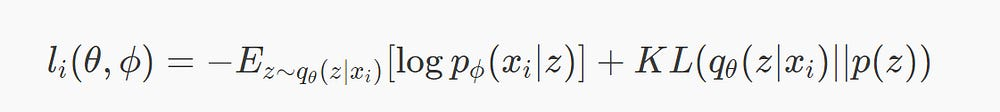Loss Function for an autoencoder. Source: http://bit.ly/2v7PBrD

#### A probabilistic perspective

This process of teasing out a mapping from input to hidden representation is called representation learning. With the Bayesian perspective, the encoder becomes a variational inference network, mapping observed inputs to (approximate) posterior distributions over latent space, and the decoder becomes a generative network, capable of mapping arbitrary latent coordinates back to distributions over the original data space .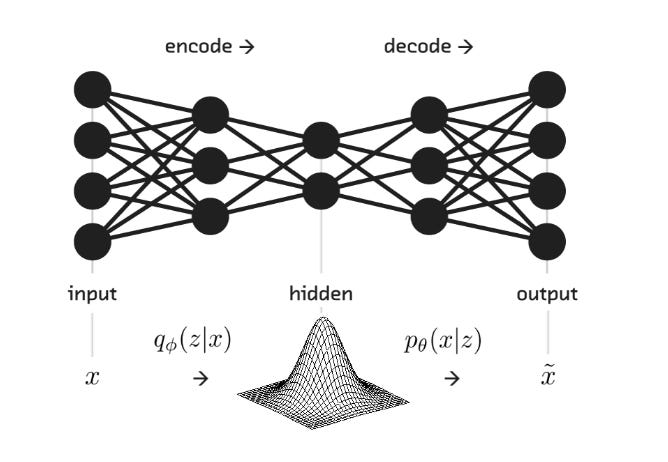Variational autoencoders. Source: 

Whereas a vanilla autoencoder is deterministic, a Variational Autoencoder is stochastic — a mashup of:

• a probabilistic encoder qϕ(z|x)
• a generative decoder pθ(x|z)

In other words, a VAE represents a directed probabilistic graphical model, in which approximate inference is performed by the encoder and optimized alongside an easy-to-sample generative decoder. These complementary halves are also known as the inference (or recognition) network and the generative network.

We can write the joint probability of the model as p(x,z)=p(xz)p(z). The generative process is the following one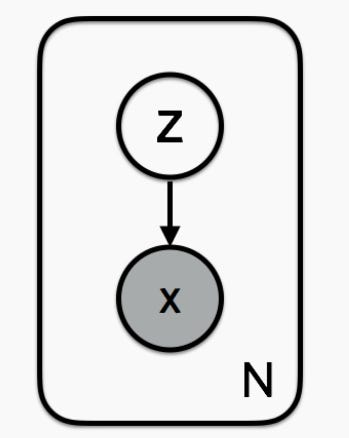Variational Autoencoder as a graph model. Source: 

The latent variables are drawn from a prior p(z). The data x have a likelihood p(xz) that is conditioned on latent variables z. The model defines a joint probability distribution over data and latent variables: p(x,z). We can decompose this into the likelihood and prior: p(x,z)=p(xz)p(z). For black and white digits, the likelihood is Bernoulli distributed.

The goal is to infer good values of the latent variables given observed data, or to calculate the posterior p(zx). According to Bayes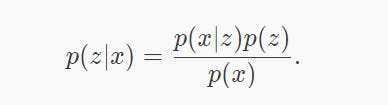Bayes for posterior probability

The denominator is called the evidence and it can be calculated by marginalizing out the latent variables

To compute this integral we need to evaluate all configurations of latent variables. As a consequence, we need to approximate the posterior distribution.

Variational inference approximates the posterior with a family of distributions qλ​​(zx). The variational parameter λ indexes the family of distributions. How can we know how well our variational posterior q(zx) approximates the true posterior p(zx)? We can use the Kullback-Leibler divergence: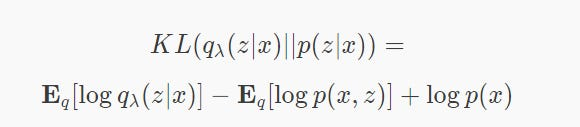Kullback-Leibler divergence

We can define ELBO (Evidence Lower BOund) as:

Minimizing the Kullback-Leibler divergence is equivalent to maximizing the ELBO and it allows us to do approximate posterior inference, what is computationally possible . For more information about variational inference you can visit .

### Resources

1. Zocca, V; Spacagna, G; Slater, D; Roelants, P. Python Deep Learning 2017
2. Intuitively Understanding Variational Autoencoders http://bit.ly/2TW3gNX
3. Tutorial — What is a variational autoencoder? http://bit.ly/2v7PBrD
4. Introducing Variational Autoencoders (in Prose and Code) http://bit.ly/2AuM89j
5. Under the hood of the Variational Autoencoder (in Prose and Code) http://bit.ly/2ABQhIq
6. Variational Inference: A review for statisticians https://arxiv.org/abs/1601.00670